FreeBuf.COM网络安全行业门户，每日发布专业的安全资讯、技术剖析。FreeBuf+小程序

2016-08-19 09:23:46

0x00 绪论

## 0x01 RSA算法简介

RSA算法又称为RSA公开密钥密码体制——所谓的公开密钥密码体制就是使用不同的加密密钥与解密密钥，是一种“由已知加密密钥推导出解密密钥在计算上是不可行的” 密码体制。RSA是被研究得最广泛的公钥算法，从提出到现在的三十多年里，经历了各种攻击的考验，逐渐为人们接受，普遍认为是目前最优秀的公钥方案之一。

RSA算法是一种非对称密码算法，所谓非对称，就是指该算法需要一对密钥，使用其中一个加密，则需要用另一个才能解密。

RSA算法涉及的参数有n、p、q 、e1、e2等。

RSA加解密的算法完全相同，设A为明文，B为密文，则：

``A=B^e1 mod n；B=A^e2 mod n；``

e1和e2可以互换使用，即：

``A=B^e2 mod n；B=A^e1 mod n;``

## 0x02 Pari简介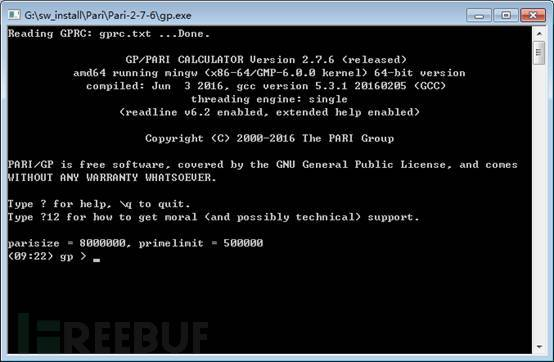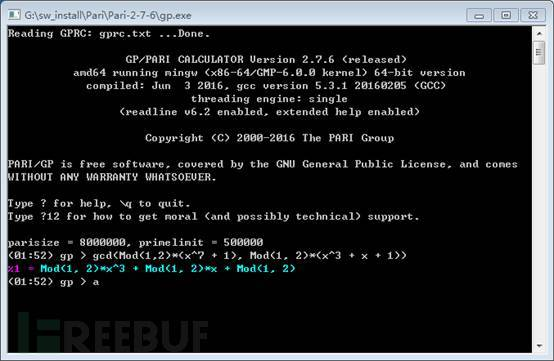## 0x03 举个栗子

e1=463884209889475377478742046340962125043690799298268667047773，

B=825568930611462903672838325065041844731508649912306161613952,

1) 给出N的分解；

2) 求私钥；

3) 求B对应的明文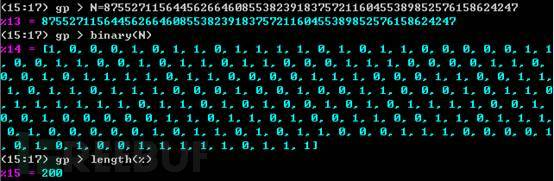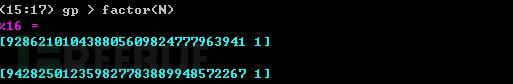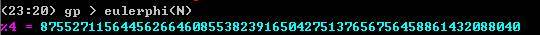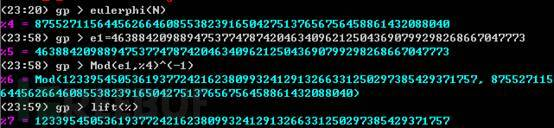``A=B^e2 mod N；B=A^e1 mod N``

``````Quick_Mod(x, n, N) =
{
bin_n= binary(n);
len= length(bin_n);
result= 1;
power_x= Mod(x, N);
for(i= 1, len,
if(bin_n[len+ 1 - i],
result*= power_x;
);
power_x= power_x^2;
);
return(lift(lift(result)));
}``````

``Quick_Mod(x, n, N)= { bin_n =binary(n); len = length(bin_n); result = 1; power_x = Mod(x, N); for(i = 1,len,if(bin_n[len + 1 - i],result *= power_x; ); power_x = power_x^2; );return(lift(lift(result))); }``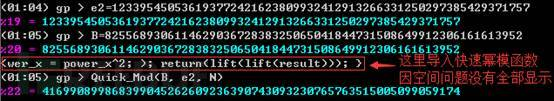*本文作者：陡壁，本文属FreeBuf原创奖励计划，未经许可禁止转载

# RSA # Pari

+ 收入我的专辑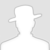• 0 文章数
• 0 评论数
• 0 关注者